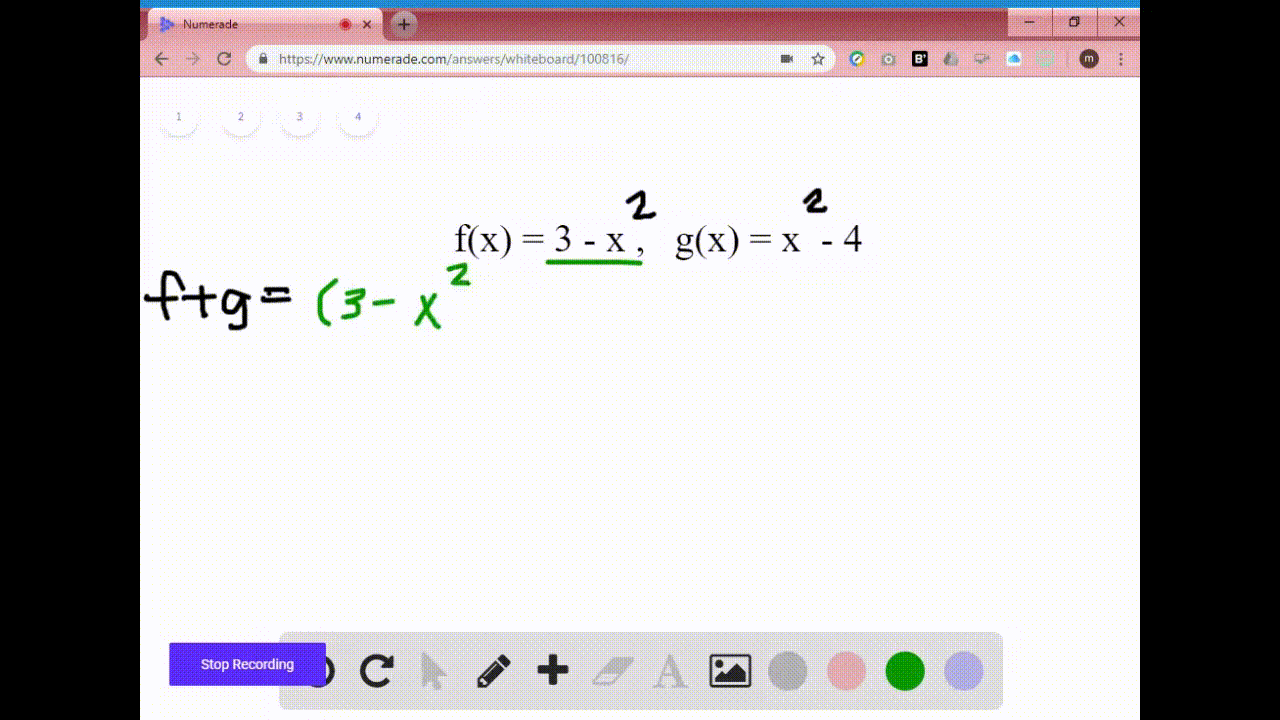Enroll in one of our FREE online STEM summer camps. Space is limited so join now!View Summer Courses## Discussion

You must be signed in to discuss.

## Video Transcript

So in this problem, we're told that F of X is equal to X squared plus X and G of X is equal to X squared. So we want to find the following combinations of these functions along with their domains. So start with F plus ci. Well, that means to take my ex function F function, which is X squared plus X, and add it to my GI function, which is X squared. Well, I can simplify this by combining my light terms. An X squared plus X squared is two ex word, so I'm left with two x squared plus x. So now for the domain well, the domain of both f n g are all real numbers because you can plug in any value you want and get a value for why? And when I combine them, I can also do the same thing. So the domain for F plus g will be negative. Infinity deposit infinity Now for F minus G, that means to take thea function, which is X squared plus X and subtracted GI function, which is minus X where Well, now we want to simplify, so we're gonna combine our white terms Well X squared minus X squared zero they canceled. So we're just left with Dex. So now for the domain. Well, our domains for F and G, we're all real numbers in the function we get when we subtract them also has a domain that's all real numbers. So the domain for F minus G is from negative. Infinity deposit infinity Now for F times G, That means to take my f function X squared plus X and times it by my GI function, which is X square. So now I can distribute to simplify this which will give us X to the fourth plus X to the third. Now we can find its domain. Well, the domain for both f n g are all real numbers and when we multiply him, we get a function whose domain is also all real numbers. So the domain for F times G is negative. Infinity deposit infinity And lastly, we have f divided by G. Well, that means to take our F function, which is X squared plus x, and we're gonna divide it by RG function, which is X square. So the only thing we can dio is each term in both the numerator and denominator can get reduced by an ex. So if I do that, I would be love with X plus One all over X now for our domain. Well, the domain of both f n g is all real numbers. However, when we do f divided by G know this how and I'm gonna always go to my first line. We have the function X square in our denominator. We have to make sure that X where will not be equal to zero. So when is X word equal to zero? Well, that happens when X is equal to zero. So that means zero cannot be in the domain for F divided by G. However, X could be anything else. So the domain for F divided by G will be negative. Infinity to zero and then from zero to infinity.